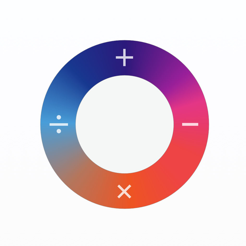## Screenshots

•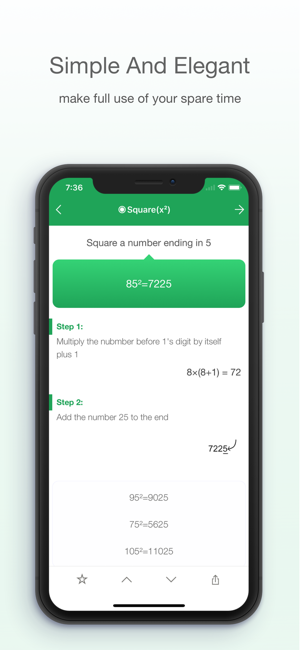•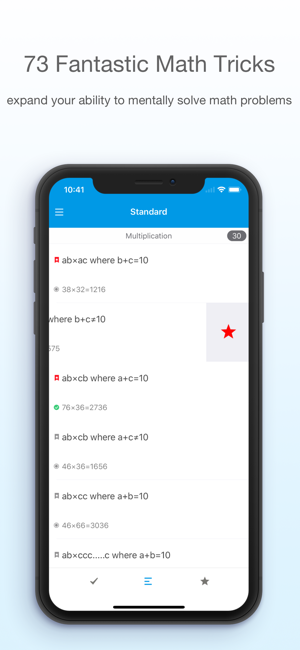••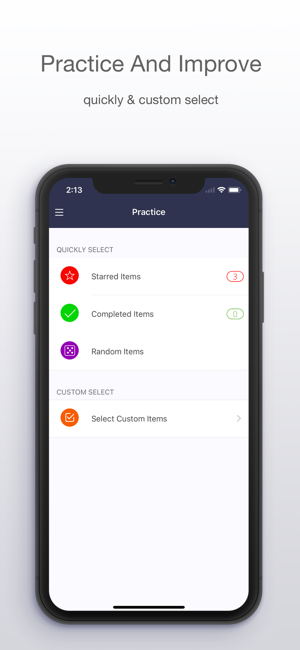•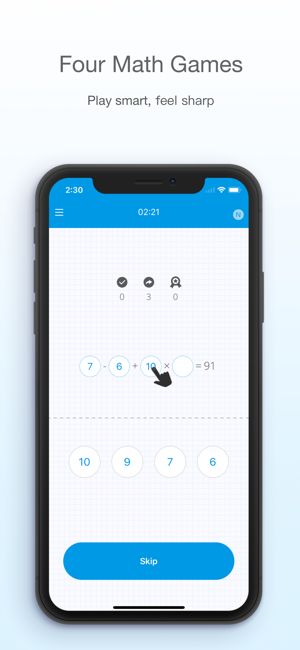## Description

Mencal is an app help you expand your ability to mentally solve math problems. All of us can do simple addition and subtraction of one or two digit numbers without a calculator.Now mencal is here to kick that ability into overdrive.

Mencal provides you with lessons and simple tricks to allow you to: work with three digit numbers; fractions of different styles regardless if you are adding them or subtracting them; and finding sums with multiplication and division. Then it will test you on your grasp and knowledge of the methods you've learned.

• Example:
- Same ten's digit,1's sum to 10
38×32=1216
①:Multiply tens place digit by itself plus 1
(3+1)×3=12
②:Add two zeros to the end of step①
12<—00=1200
③:Multiply the two one's digits together
8×2=16
④:Add the results of step ②and③
1200+16=1216

• Quickly Square:
- Square numbers made up of 1's
- Square numbers made up of 3's
- Square numbers in the 90's
- Square numbers ten`s digit is 0
- Square a number ending in 25
- Square numbers made up of 6's
- Square numbers made up of 9's
- Square a number ending in 1
- Square a number ending in 5
- Square a number ending in 9
- Square numbers in the 10's
- Square numbers in the 40's
- Square numbers in the 50's
- Square numbers in the 500's
- Square numbers in the 600's
- Square numbers in the 700's
- Square numbers in the 800's
- Square numbers in the 900's

•Standard:
- Same ten's digit, 1's sum is 10
- Same ten's digit, 1's sum isn`t 10
- Same 1's digit, ten's digits sum is 10
- Same 1's digit, ten's digits sum isn`t 10
- two-digit number ab×cc (a+b=10)
- ab×ccc.....c (a+b=10)
- Multiply by 11
- 2-digit number if 1's digit is 1
- 2-digit number if tens place digit is 1
- 2-digit number ×2-digit number(universal)
- Multiply differing by 5
- Multiply differing by 6
- Multiply differing by 7
- Multiply differing by 8
- Multiply differing by 9
- Multiply differing by 10
- Multiply two numbers that in the 90‘s
- Two-digit number like ab+ba
- Three-digit number like abc+cba
- Two fractions like 1/a+1/b
- Two fractions like (a-1)/a + (b-1)/b
- Sum of first n odd numbers
- Sum of first n even numbers
- Two-digit number like ab-ba
- Three-digit number like abc-cba
- m-n if m+n=100,1000,10000...
- Two fractions like 1/a-1/b
- Two fractions like c/a-c/b
- Two fractions like (a-1)/a-(b-1)/b
- Divide any number by 5
- Divide any number by 25
- Divide any number by 125
- Divide any 3-figure number by 9

"This app rocks!! Great for Math Team practice!" -by Bubba Smith
"Great app, make me feel less stupid" -by Howie_Serious
"Its a useful app for anyone who wants to know the numbers deeper" -by sjzbim
"It's a good app for both kids and sometimes even adults" -by Willwang

Love mencal or have a question? We would love to hear your feedback! Contact us anytime at instantcj@gmail.com

## What’s New

Version 3.70

● Additional bug fixes and stability improvements
● Remove math game In-App purchase

## Ratings and Reviews

5.0 out of 5
4 Ratings

4 Ratings

Donn1hew ,

### Great App

You just have to devote time do the application and do examples over and over and over again. With time your master these exercises. Your mastery will save you a lot of time.

はなちやん@.@ ,

### Magical experience !

I've definitely been enlightened after merely a few minutes usage of this app. Right now I've been captured by the unfathomably mesmerizing beauty of this app. Try it you won't regret.

Ecp84 ,

### Could be great

This is the best-looking app of this sort that I have seen (other than the choice of handwritten/chalkboard font for the numbers), but something tells me the appearance took precedence over the importance of utility. The instructions for methods are sometimes unclear, and not only because it looks as though the person who wrote it was not a native English-speaker. Sometimes crucial words are omitted. Sometimes it's just unclear what's being said.
Additionally, it is my opinion that the app could use some expansion regarding how one is able to test oneself. Let the user test him- or herself incrementally with each step as they begin to understand it, or allow the user to include or omit the methods used in the testing portion. Otherwise, it requires the user to master and understand each kind of method before feeling they can challenge themselves effectively. Otherwise, this is a great app, and a beautiful one to boot. I'd love to use this more often if I could seem to have it work more in my favor as I learn and test myself with each method. It certainly had great potential, and I look forward to seeing how/if it changes in the future.

## Information

Seller
Jun Cao
Size
9.7 MB
Category
Education
Compatibility

Requires iOS 8.0 or later. Compatible with iPhone, iPad, and iPod touch.

Languages

Age Rating
4+
Price
\$1.99
In-App Purchases
1. Unlock all math games \$0.99
2. Unlock Numbers Mode \$0.99
3. Unlock Operators Mode \$0.99

## Supports

•### Game Center

#### Challenge friends and check leaderboards and achievements.

•